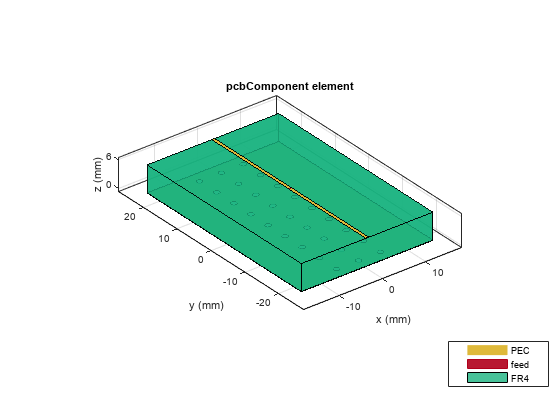# Board Thickness versus Dielectric Thickness in PCB

This example shows you how to define the BoardThickness of PCB with respect to dielectric thickness of `pcbComponent `object for different use-cases.

The BoardThickness is the property of `pcbComponent` object and its value is the sum of thicknesses of all the dielectric layers that lie below the top metal layer. Dielectric layers above the top metal layer are considered as coating and not included in the BoardThickness calculations.

Note: Define BoardThickness before defining the Layers.

The three different sections defines the board thickness for different use-cases:

1. Section one defines the BoardThickness for a single-layered dielectric PCB.

2. Section two defines the BoardThickness for a multi-layered dielectric PCB and

3. Section three defines the BoardThickness when importing the PCB using a `pcbReader `object.

### Single-Layered dielectric pcbComponent

In this use case, the PCB a single dielectric layer sandwiched between the top metal layer and bottom metal layer. Here, h defines the BoardThickness and the d is the dielectric thickness.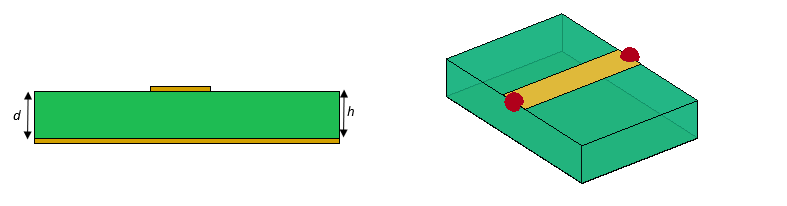When the pcbComponent has a single dielectric layer, the BoardThickness (h) should be equal to dielectric thickness (d). If h` `is not equal to d`, `then d is updated to match h.

```p = pcbComponent; sub = dielectric('Fr4'); TopMetal = p.Layers{1}; BtmMetal = p.Layers{3}; p.BoardThickness = sub.Thickness; p.Layers = {TopMetal,sub,BtmMetal}; figure; show(p);```### Multi-Layered dielectric pcbComponent

#### The dielectric layers are present below the top metal layer.

For multi-layered dielectrics in a PCB, the BoardThickness will be the sum of thickness of the dielectric layers below the top metal layer. The dielectrics above the top metal layers are considered as a coating and will not be included in the board thickness calculations.

In this use case, there are two dielectric layers below the top metal layer and one dielectric layer below the bottom metal layer as shown in the figure below. The BoardThickness (h) will be the sum of dielectric layers below the top metal layer (i.e. h = d1+d2+d3).```p = pcbComponent; sub1 = dielectric('FR4'); sub2 = dielectric('Teflon'); TopMetal = p.Layers{1,1}; BtmMetal = p.Layers{1,3}; % Set the BoardThickness as sum of dielectric thickness below the top metal % layer h = 2*sub1.Thickness+sub2.Thickness; p.BoardThickness = h; p.Layers = {TopMetal,sub1,sub2,BtmMetal,sub1}; p.FeedLocations(:,3:4) = [1,4;1,4]; figure; show(p)```In this use case, there is a dielectric layer present above the top metal layer and air dielectric separates the top and bottom metal layers. Add another dielectric layer below the bottom metal layer. The dielectric layer which is present above the top metal layer is considered as coating and is included in the BoardThickness calculation. The BoardThickness (h) will be the sum of dielectric layers below the top metal layer (ie. h= d2+d3).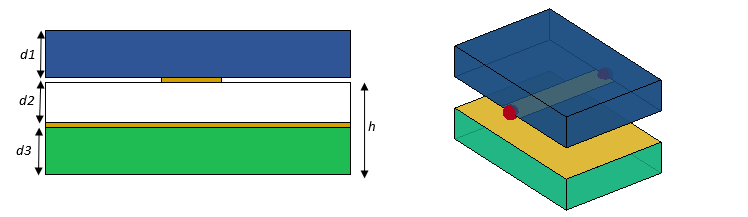```p = pcbComponent; sub1 = dielectric('FR4'); sub2 = dielectric('Air'); sub3 = dielectric('Teflon'); TopMetal = p.Layers{1,1}; BtmMetal = p.Layers{1,3}; % Set the BoardThickness as sum of dielectric thickness below the top metal % layer h = sub2.Thickness+sub3.Thickness; p.BoardThickness = h; p.Layers = {sub1,TopMetal,sub2,BtmMetal,sub3}; p.FeedLocations(:,3:4) = [2,4;2,4]; figure; show(p)```#### The dielectric layers are present only above the top metal layer

In this use case, PCB has multilayer dielectrics that are above the top metal layer. The BoardThickness(h) will be the sum of thickness of all the dielectric layers (h = d1+d2).

Note: This is applicable only when there are no dielectrics present below the top metal layer.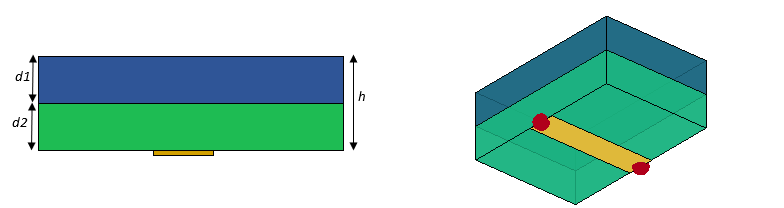```p = pcbComponent; sub1 = dielectric('FR4'); sub2 = dielectric('Teflon'); TopMetal = p.Layers{1,1}; % Set the BoardThickness as sum of dielectric thickness below the top metal % layer h = sub1.Thickness+sub2.Thickness; p.BoardThickness = h; p.Layers = {sub1,sub2,TopMetal}; p.FeedLocations(:,3:4) = 3; figure; show(p);```### Create pcbComponent for pcbReader workflow

Import the top and bottom layers from the Gerber file by setting .gtl and .gbl file to Layer2 and Layer4 in `stackUp` function. Pass the stackUp object to the `PCBReader `object.

```S = stackUp; S.Layer2 = 'EBGstruct.gtl'; S.Layer4 = 'EBGstruct.gbl'; p = PCBReader ('StackUp',S);```

When the PCBReader object is converted as pcbComponent , the pcbComponent will read all the 5 layers of stackUp object, where air dielectric is the default top and bottom layer. This is a multilayered dielectric use case. The BoardThickness will be the sum of thickness of dielectric below the top metal layer (ie. h = d2+d3).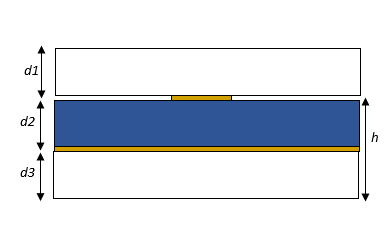```pcb = pcbComponent(p); pcb.BoardThickness = pcb.Layers{3}.Thickness+pcb.Layers{5}.Thickness; pcb.FeedLocations = [0,0,2]```
```pcb = pcbComponent with properties: Name: 'EBGstruct' Revision: 'v1.0' BoardShape: [1x1 antenna.Rectangle] BoardThickness: 0.0061 Layers: {[1x1 dielectric] [1x1 antenna.Polygon] [1x1 dielectric] [1x1 antenna.Polygon] [1x1 dielectric]} FeedLocations: [0 0 2] FeedDiameter: 0.0100 ViaLocations: [] ViaDiameter: [] FeedViaModel: 'square' Conductor: [1x1 metal] Tilt: 0 TiltAxis: [0 0 1] Load: [1x1 lumpedElement] ```
```pcb.FeedDiameter = 0.1e-3; show(pcb);```Modify the above use case by removing the top and bottom air dielectric layers as show in figure. This use case is now a single-layer dielectric pcbComponent. Now you can change the dielectric thickness by changing the BoardThickess.(h = d).```pcb = pcbComponent(p); d = pcb.Layers{3}; pcb.BoardThickness = d.Thickness; L = {pcb.Layers{2},pcb.Layers{3},pcb.Layers{4}}; pcb.Layers = L; pcb.FeedLocations = [0,0,1]; pcb.FeedDiameter = 0.1e-3; show(pcb);```